# ISEE Upper Level Quantitative : How to find if triangles are congruent

## Example Questions

### Example Question #13 : Acute / Obtuse Triangles

Given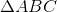and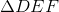with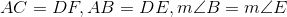Which is the greater quantity?

(a)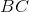(b)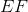(a) is greater.

(b) is greater.

(a) and (b) are equal.

It is impossible to tell from the information given.

It is impossible to tell from the information given.

Explanation:

Examine the diagram below, in which two triangles matching the given descriptions have been superimposed.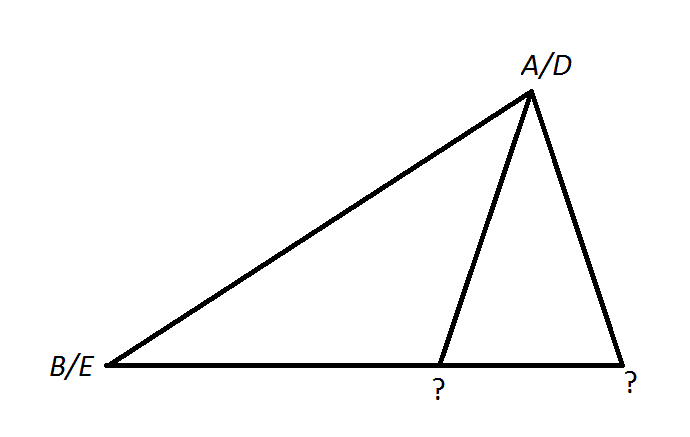Note that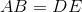and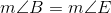. The two question marks need to be replaced byand. No matter how you place these two points,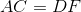. However, with one replacement,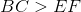; with the other replacement,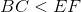. Therefore, the information given is insufficient to answer the question.

### Example Question #14 : Acute / Obtuse Triangles

Considerandwith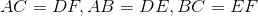.

Which is the greater quantity?

(a)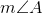(b)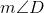(a) is greater.

It is impossible to tell from the information given.

(b) is greater.

(a) and (b) are equal., so, by the Side-Side-Side Principle, since there are three pairs of congruent corresponding sides between the triangles, we can say they are congruent - that is,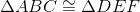.
Corresponding angles of congruent sides are congruent, so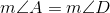.